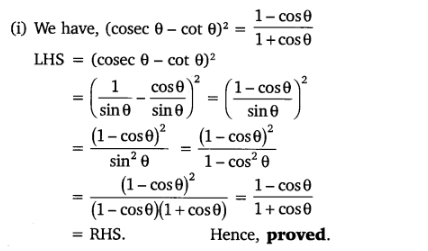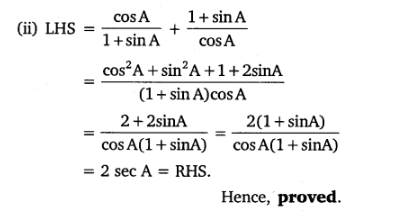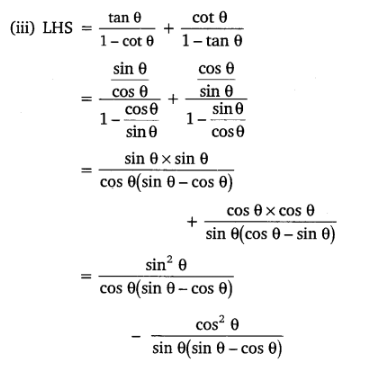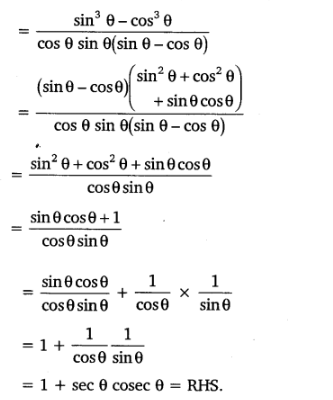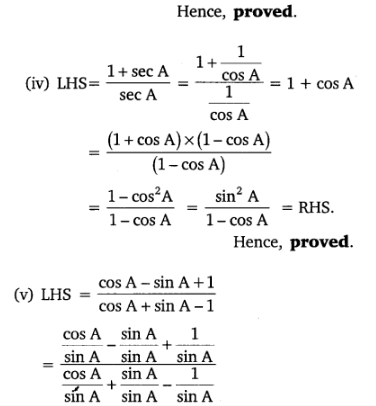• +91 9971497814
• info@interviewmaterial.com

# Introduction to Trigonometry Ex-8.4 Interview Questions Answers

### Related Subjects

Question 1 :

Express thetrigonometric ratios sin A, sec A and tan A in terms of cot A.

Answer 1 :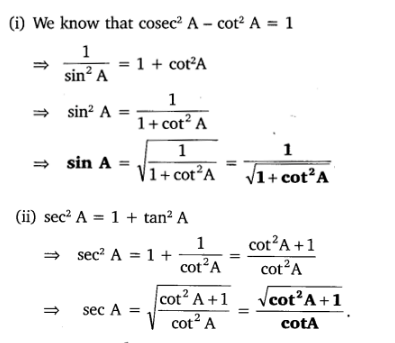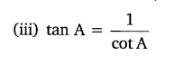Question 2 : Write all the other trigonometric ratios of A in terms of sec A.

Answer 2 :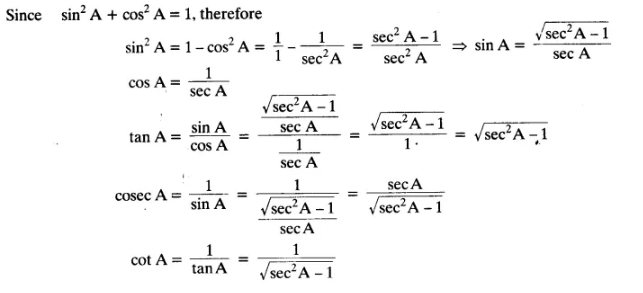Question 3 :

Evaluate:

(i) (sin263°+ sin227°)/(cos217° + cos273°)
(ii)  sin 25° cos 65° + cos 25° sin 65°

Answer 3 :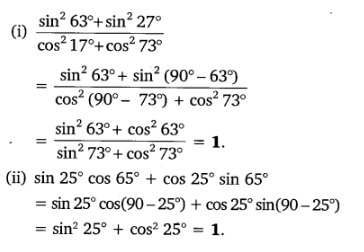Question 4 : Choose the correct option. Justify your choice.

(i) 9 sec2A – 9 tan2A =
(A) 1                 (B) 9             (C) 8               (D) 0
(ii) (1 + tan θ + sec θ) (1 + cot θ – cosec θ)
(A) 0                 (B) 1             (C) 2               (D) – 1
(iii) (sec A + tan A) (1 – sin A) =
(A) sec A           (B) sin A       (C) cosec A      (D) cos A

(iv) 1+tan2A/1+cot2A=

(A) secA                (B) -1             (C) cot2A               (D) tan2A

Solution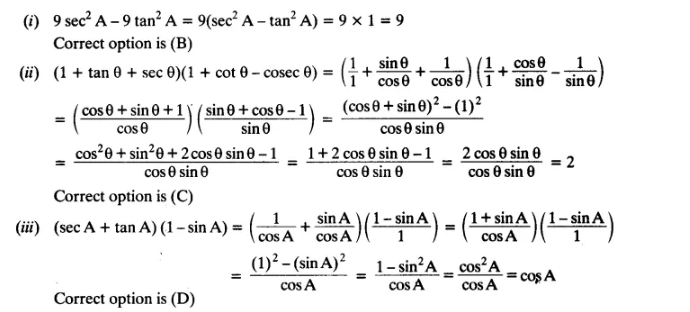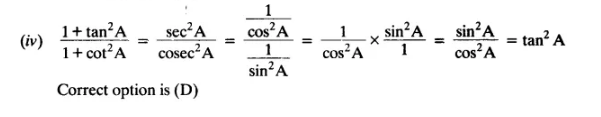Question 5 :

Prove the followingidentities, where the angles involved are acute angles for which the
expressions are defined.

(i) (cosec θ – cotθ)= (1-cos θ)/(1+cos θ)

(ii) cos A/(1+sinA) + (1+sin A)/cos A = 2 sec A

(iii) tan θ/(1-cotθ) + cot θ/(1-tan θ) = 1 + sec θ cosec θ

[Hint : Write the expression in terms of sin θ and cos θ]

(iv) (1 + secA)/sec A = sin2A/(1-cos A)

[Hint : Simplify LHS and RHS separately]

(v) ( cos A–sinA+1)/( cos A +sin A–1) = cosec A + cot A, using the identity cosec2A= 1+cot2A.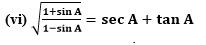(vii) (sin θ – 2sin3θ)/(2cos3θ-cosθ) = tan θ
(viii) (sin A + cosec A)+ (cos A + sec A)2 = 7+tan2A+cot2A
(ix) (cosec A – sin A)(sec A – cos A) = 1/(tan A+cotA)
[Hint : Simplify LHS and RHS separately]
(x) (1+tan2A/1+cot2A) = (1-tan A/1-cot A)2 = tan2A

Solution